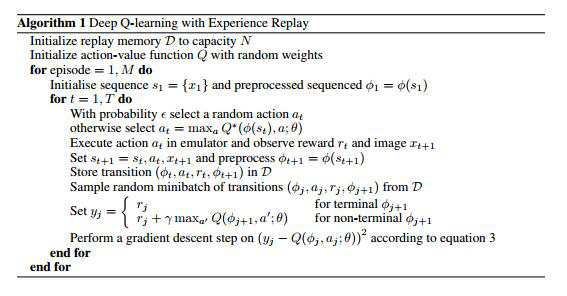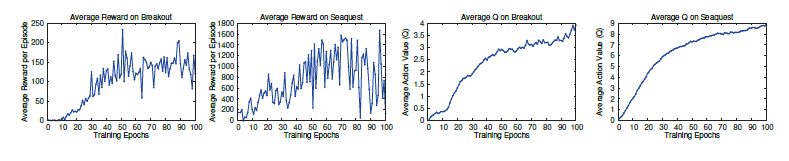# 单智能体强化学习算法

## DQN：Playing Atari with Deep Reinforcement Learning

Posted by MY on August 3, 2020

## 一、问题

• 针对不同的游戏需要提取不一样的feature。对于打砖块可以提取上面提到的球的位置，挡板的位置，剩下砖块的位置等。但是换了一个游戏，比如flappy bird，你就需要花很多时间想办法获取小鸟的位置以及各个水管的位置等。这会导致一个游戏的算法不能很好的应用在另一个游戏上，没有实现通用性。
• 提取的feature很复杂的时候，会造成存储或计算的瓶颈（状态空间过大）。还是以打砖块为例，提取游戏中的feature的时候，如果直接提取位置，假设以像素作为坐标表示，我们需要提取的feature就会由很多坐标组成，然后每个坐标又有很多情况，这样就会造成我们提取的feature可能的情况太多，使用传统的Qlearning的话需要为每个state估计Qvalue，直接造成计算量过大，并且可能无法存下这么巨大的Qtable。
• 提取的feature不一定准确。为了防止提取的feature过于复杂，我们往往会对feature进行压缩，找出关键性的feature喂给RL模型进行学习。比如flappy bird中我们可以把小鸟的位置和各个水管的位置改成小鸟和下一个水管的相对位置等。这样虽然可以将复杂的feature简化，从而减小状态空间的大小。但是存在的问题是我们提取到的feature不一定准确。我们提取feature的时候往往受限于自身经验，对于简单问题也许还好，只要仔细并且实验几次往往不会有问题，但是对于复杂的问题，人类经验显得不足或者是错误的时候，这样提取的feature肯定不会达到很好的效果。

## 二、解法

### 2.2 迭代公式推导

$R_{t}=r_{t}+r_{t+1}+r_{t+2}+\ldots+r_{T}$

$R_{t}=r_{t}+\gamma * r_{t+1}+\gamma^{2} * r_{t+2}+\ldots+\gamma^{T-t} * r_{T}$

\begin{aligned} R_{t} &=r_{t}+\gamma * r_{t+1}+\gamma^{2} * r_{t+2}+\ldots+\gamma^{T-t} * r_{T} \\ &=r_{t}+\gamma *\left(r_{t+1}+\gamma * r_{(t+1)+1}+\ldots+\gamma^{T-(t+1)} * r_{T}\right) \end{aligned}

\begin{aligned} Q^{*}\left(s_{t}, a_{t}\right) &=R_{t} \\ &=r_{t}+\gamma *\left(r_{t+1}+\gamma * r_{(t+1)+1}+\ldots+\gamma^{T-(t+1)} * r_{T}\right) \end{aligned}

\begin{aligned} Q^{*}\left(s_{t}, a_{t}\right) &=r_{t}+\gamma *\left(r_{t+1}+\gamma * r_{(t+1)+1}+\ldots+\gamma^{T-(t+1)} * r_{T}\right) \\ &=r_{t}+\gamma * \max _{a^{\prime}} Q\left(s_{t+1}, a^{\prime}\right) \end{aligned}

### 2.3 算法• 每一步的经验可能在更新神经网络权重的时候被使用很多次，让数据使用更有效率。
• 由于连续的样本之间有很强的关联性，使用连续的样本比较没有效率。而随机的样本能打破这种关联
• 如果不使用experience replay而采用on-policy的机制，当前的参数就决定了下一个训练样本，我们又要根据这个样本训练我们的参数，这样很容易导致训练过程中不愿意看到的反馈回路(feedback loop)，结果可能导致收敛到了一个局部最优解甚至是结果产生灾难性的偏移。

## 三、实验内容## 五、优点

##### Share